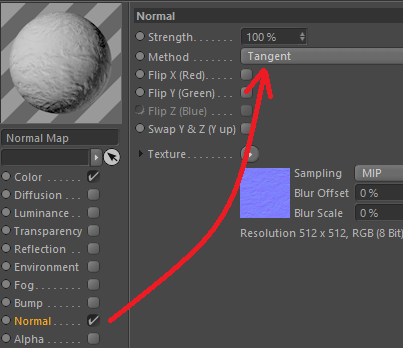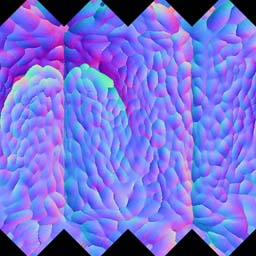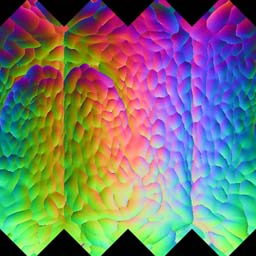# Solved Normal tangents to world

Hi folks,

I'm trying to meddle with normal maps by converting them to local (and then world) space. I'm 'printing' the map to the Picture Viewer to see the result.

But I'm not grasping how to change from normal tangent to local space. I've read a bunch of opengl stuff but it's not helping.

``````// pseudo code

Matrix m = Get_ObjectRotMat(); // I've got this
Vector normal = Get_PolyNormal(); // I've got this
Vector norm_tang_texture = Get_NormalMapValue(); // I've got this

/* I can't seem to do this */
Vector norm_tang_to_local =  ??;

/* I can do this, but is useless without the above? */
Vector local_to_world = etc.;
``````

Would someone be so kind as to give me a lesson on this?

WP.

Hello @wickedp,

Thank you for reaching out to us. We will answer this question tomorrow more thoroughly, you happend to post after our morning meeting, but I can already see some questions we might have.

But I'm not grasping how to change from normal tangent to local space.

I am a bit confused with what you mean by normal tangent space. I assume you are referring to tangent space for a normal map. A normal map is normal (deviation) information in the space of the object it is applied to.

`Vector norm_tang_to_local = ??;`

The question is what you expect here to happen. Do you want to sample by a texture coordinate, then you can just use `BaseShader::Sample()`. Or do you want to sample by a point in world or local object space and you want to find the closest normal (driven by a normal map or not)? Your code sort of implies that you want to use your normal information called `normal`. Then you must simply apply the normal map sample to the vertex normal. I am assuming here that the return value of `Get_NormalMapValue()` is already a normal, if not, see Normal Mapping for how to convert from an RGB vector to a normal. As pseudo code:

``````// The vertex to sample.
const maxon::Int32 vertextIndex = 0;

// Your function, not required, everything is in object space.
const Matrix m = Get_ObjectRotMat();

// Your function, I am using this as if it would return a vertex normal.
const Vector vertex_normal = Get_PolyNormal(vertextIndex);

// Convert a coordinate in object space to texture space. This is IMHO the tricky
// part, your code implies that you got this, but it is quite hard to do IMHO.
const Vector uvw = GetTextutureCoordinate(mesh, vertextIndex);

// Your function, this has its problems as explained below. I am also using this as if
// it would sample at a point and not an area.
const Vector texture_normal = Get_NormalMapValue(uvw);

// Builds a frame, i.e., a rotation matrix/transform for the given texture normal.
const Matrix normal_transform = BuildNormalFrame(texture_normal, upVector);

// The final vertex normal tranformed by the normal map.
const Vector final_normal = normal_transform * vertex_normal;
``````

Where `BuildNormalFrame` is a function which builds a frame for a vector in the common way with an input vector, an up-vector and the cross product. When the normal map is purple, the normal deviation zero, `texture_normal` will be the null vector and you should bail on doing further computations or just forcibly set `normal_transform` to the identity matrix. `final_normal` will then be the vertex normal rotated by the orientation stored in the normal map. E.g., when the RGB value was `(128, 0, 128)`, i.e., the texture normal is `(0, -1, 0)` (as opposed to the 'default' value of `(0, 0, 1)` for the normal/z/k/v3 axis of a transformation matrix/frame). If then the vertex normal is `(1, 0, 0)`, the final normal is `(0, 0, 1)`, i.e., the vertex normal rotated 90° CCW on the Y-axis.

There is also the problem of texture sampling, which is not trivial. Your posting says `Get_NormalMapValue(); // I've got this`. But I would consider this the tricky part, since you will have to sample the texture at the location of the vertex which has the normal `normal`. The function you are using to retrieve the geometry normal is also called `Get_PolyNormal()`, which implies an area to be sampled and not a (vertex) point. So, `Get_NormalMapValue()` also samples and interpolates an area in the normal map and then returns that value? This is all quite unclear to me. There is `VolumeData::GetWeights()` which gives you exactly the algorithm Cinema 4D is using for retrieving a texture sample. But using this requires render-time only information. The next best option is maxon::GeometryUtilsInterface which also contains methods to convert from Cartesian (a vertex position in object space) to Barycentric coordinates (a texture coordinate). The problem is that this works on triangle level, so the uv(w) texture coordinate you get out of it is not the one you get for a whole mesh. Redoing texture sampling outside of the rendering pipeline is hard.

If you just want to get what the Cinema 4D normal map functionalities produce, you could also use texture baking.

Cheers,
Ferdinand

edit: I updated the posting.

MAXON SDK Specialist
developers.maxon.net

Thanks Ferdinand,

I was writing a reply when your edit came through. I'll post what I was typing, but bear with me while I think about your updated edits.

/* Original message */

no need to rush, all good! I'm trying to get in my head what I'm wanting to ask. I'll try with some examples.

Normal maps can be in different spaces. I'm using the term 'spaces' loosely, but I'm referring to this:There are three types of normal maps we can have. Tangent, Object and World.

For example, let's say I run a mouse click in the viewport. I can capture all the information I need, like the hit object, hit polygon, the UV texture coords, etc etc and also have the phong shaded normal (in either object or world space). Let's say we have all the bits needed to do all of that.

Now I sample the normal texture map using the UV coordinates and get the normal texture map value at the sampled location. If the sampled texture map value is in tangent 'space', how can I change it to object 'space'?

I want to go from this (tangent):To this (object):How can I translate the texture map tangent-based texel, to object 'space'?

WP.

Hey @WickedP,

How can I translate the texture map tangent-based texel, to object 'space'?

This is what I have lined out above. A normal map is information for how the normal of a geometry of at the point `p` should change. For that you must construct a transform for the normal stored in the texture map as lined out above, i.e., define the remaining two vectors of a basis (the tangent and bi-normal). And then simply transform the vertex normal with that. Which is why the raw normal map looks sort of 'unicolor', as it only stores deviation information, while a final texture for a specific object looks 'colorful', as it also includes specific geometry information, the vertex normals multiplied by the normal map normals (and the interpolation between these 'final' normal). The tricky part (in Cinema 4D) is how to sample a texture applied to a mesh at a coordinate in object space, e.g., a vertex position. I would not know a good way to do this outside of the rendering process without having to reinvent the wheel of texture sampling.

Which also makes it problematic to provide example code, because

Vector norm_tang_texture = Get_NormalMapValue(); // I've got this

at least I have not covered this so easily.

If this is not for academic purposes, I would really point to texture baking again, since there is a lot to do here. Especially when you not only want transformed vertex normals, but also an output texture which interpolates between these vertices and maps everything back to uvw space.

Cheers,
Ferdinand

MAXON SDK Specialist
developers.maxon.net

Hi Ferdinand,

What's in this, what does this look like inside:

// Builds a frame, i.e., a rotation matrix/transform for the given texture normal.
const Matrix normal_transform = BuildNormalFrame(texture_normal, upVector);

WP

Hello @wickedp,

What's in this, what does this look like inside:

Builds a frame, i.e., a rotation matrix/transform for the given texture normal.

Where `BuildNormalFrame` is a function which builds a frame for a vector in the common way with an input vector, an up-vector and the cross product.

I do not think that we have a C++ example for that, at least I do not know one (and I also asked @m_adam who knows them best). There is however the Python Manual for matrices, where I did cover that topic under the section Constructing and Combining Transforms. The last example shows how to construct a transform from an input vector and an up-vector.

When you need help with translating the example, let me know. I would also recommend refreshing your knowledge of linear algebra and especially a basis, i.e., a linear transform and what is represented by `Matrix` in Cinema 4D, when you feel a bit shaky on the subject. I do not want to be rude here, but it will help you to do whatever you want, and you do not have to rely on copying someone else's code.

I hope this helps and cheers,
Ferdinand

MAXON SDK Specialist
developers.maxon.net

Hello @wickedp,

without any further activity before Wednesday, the 16.03.2022, we will consider this topic as solved and remove the "unsolved" state from this topic.